# Electronics and Communication Engineering - Exam Questions Papers

21.

For the circuit, let us assume that R = 1 Ω, L = 10-3 H, and V(t) = 10 cos (1000t + 30°)V and the differential equation for I(t) is given by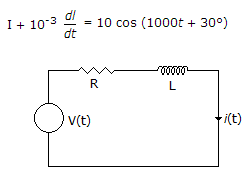A. 7.07 cos (1000t + 15°) A B. 70.7 cos (1000t - 30°) A C. 7.07 cos (1000t - 15°) A D. 7.07 cos (1000t + 15°) A

Answer: Option C

Explanation: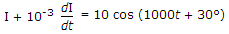Replacing current and voltage by their phasors I and 10ejp/b, resp. and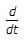by jω = j1000, we obtain the phasor equation

I + 10-3(j1000I) = 10ejp/6

or I(1 + j1) = 10ejp/6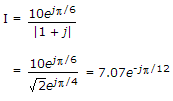Having determined the value of I, we now find the required solution to be

I(t) = Rc [I ejωt] = Rc[7.07e -jp/2 ej1000t]

= 7.07 cos (1000t - 15°)A.

22.

Find equivalent capacitance if each capacitance is 1 F.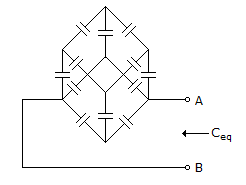A. 56 F B. 6/5 F C. 6/10 F D. 5/12 F

Answer: Option B

Explanation:

Use similar method. But for capacitor take inverse of value of capacitance and then apply procedure same as in resistance case. After getting the final answer take inverse of result to get Ceq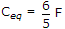In Cuboid case,

For resistance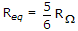For inductance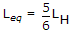For capacitance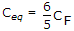.

23.

The Z inverse of the given Z transform is :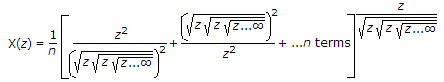A. unit step B. unit impulse C. unit ramp D. unit parabola

Answer: Option B

Explanation: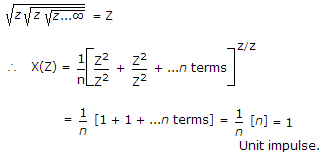24.

The RMS value of the signal given below is: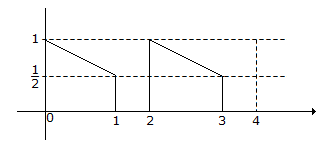A.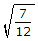B.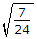C.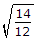D.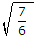Answer: Option B

Explanation:

RMS value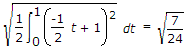.

25.

Use block diagram reduction methods to obtain the equivalent T.F from R to C.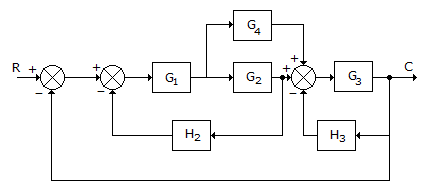A.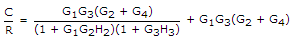B.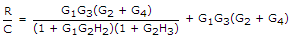C.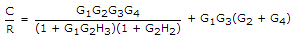D.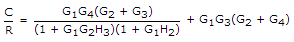Answer: Option A

Explanation: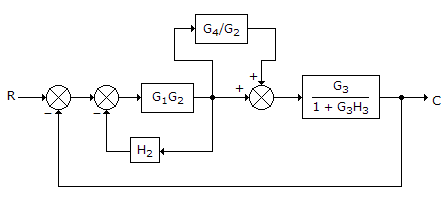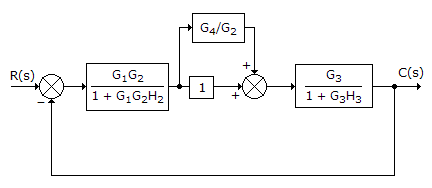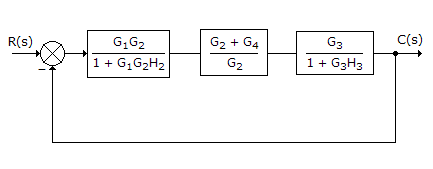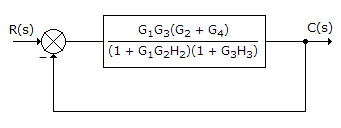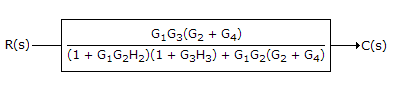#### Current Affairs 2021

Interview Questions and Answers